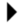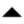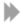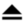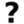## 4.3 Embedding example

Suppose you want to write a function which returns sqrt(r^2-x^2), with some of the nice features of the abs function, to be called rtleg for “right triangle leg”. Your first option is to simply write an interpreted function:

 ```func rtleg(r,x,..) { rr = 1./r; x *= rr; /* prevent overflow for very large or small r */ y = 1. - x*x; while (!is_void((x=next_arg()))) { x *= rr; y -= x*x; } return r*sqrt(y); } ```

This interpreted function is likely the best solution, because the required function is simple and vectorizes naturally. For the sake of argument, however, suppose that you wanted to create a compiled version of rtleg instead. Now you need not only interpreted code, in a file ‘rtleg.i’, but also compiled code in a file ‘rtleg.c’. Let’s consider two cases: First, let’s use the PROTOTYPE comment and let codger generate the required wrapper code. Second, let’s write the wrapper code using ‘yapi.h’ directly.